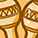cancel
Showing results for
Did you mean:

# Characteristics of Quantum DotsContributor
0 0 105

Quantum dots, also known as semiconductor nanocrystals, are nanoscale materials composed of a small number of atoms. The number of atoms in a quantum dot is usually between a few and a few hundred, and their size in all three dimensions are less than 100 nm. The movement of carriers in the three dimensions of quantum dots is limited by the size effect. Due to the quantum confinement effect, the energy levels of carriers in quantum dots are similar to those of atoms having a discontinuous energy level structure, so quantum dots are also called artificial atoms. Due to their special energy level structure, quantum dots exhibit unique physical properties. This paper mainly discusses some properties of quantum dots, including quantum confinement effect, quantum size effect, surface effect and luminescence property.

## Quantum confinement effect

Generally, the smaller the volume, the greater the bandwidth, so the optical and electrical properties of the quantum dots are highly dependent on the size of the material. Generally, when the size of the quantum dot is equal to or smaller than the exciton Bohr radius of the corresponding bulk material, the movement of the carrier electron-hole pair is in a strongly restricted state. When the energy gap increases as the particle size becomes smaller, the semiconductor material is quantified. The energy after quantification of the semiconductor material is: E(R)=Eg+h²?²/2uR²-1.8/?R. In the formula, Eg is the bulk band gap, u is the mass of electrons and holes,? is the dielectric constant of the quantum dot material, R is the radius of the particle, and E(R) is the lowest excitation energy. The value obtained by subtracting Eg from E(R) is the amount of increase in kinetic energy.

## Quantum size effect

It can be seen from the above formula that the quantum confinement energy and the coulomb interaction energy are proportional to 1/R2 and 1/R, respectively, the former can increase the band gap energy (blue shift), and the latter can reduce the band gap energy (red shift). When R is small, the quantum confinement can be more sensitive to R. As R decreases, the quantum confinement energy increases more than the Coulomb interaction energy, resulting in a blue shift of the spectrum.

## Surface effect

Surface effect means that the specific surface area of quantum dots increases with the decrease of particle size, resulting in insufficient coordination of surface atoms and increased number of unsaturated bonds and dangling bonds, thus the atoms on the surface of quantum dots are extremely unstable and easily bind to other atoms. This surface effect gives the quantum dots a large surface energy and high activity, which not only causes changes in the atomic structure of the quantum surface, but also causes changes in the surface electron energy spectrum. Surface defects lead to trapped electrons or electron holes, which in turn affect the luminescent properties of quantum dots, causing nonlinear optical effects.

## Luminescence property

The principle of luminescence of quantum dots is similar to that of conventional semiconductor luminescence, that is, carriers in a material reach an excited state after receiving external energy, and release energy when carriers return to the ground state, and this energy is usually released in the form of light. Unlike conventional luminescent materials, the luminescent materials of quantum dots have the following characteristics.